# Limits Continuity Differentiability And Differentiation Set-6

Go back to  'SOLVED EXAMPLES'

Example – 15

If    $$f(xy) = f(x) + f(y)$$  for all x, y > 0 and $$f(x)$$  is differentiable at x = 1, then prove that $$f(x)$$ is differentiable for all x > 0

Solution:   Substituting $$x = y = 1,$$   we get $$f(1) = 0$$

Since   $$f(x)$$  is differentiable at $$x = 1,f'(1)$$  exists

\begin{align}f'(1) = \mathop {\lim }\limits_{h \to 0} \frac{{f(1 + h) - f(1)}}{h}\end{align}

\begin{align}= \mathop {\lim }\limits_{h \to 0} \frac{{f(1 + h)}}{h}\,\,\,\,\,\,\,\,\,\,\,\,\,\,\,\,\,\,\, \ldots (i)\end{align}

Now, \begin{align}f'(x) = \mathop {\lim }\limits_{h \to 0} \frac{{f(x + h) - f(x)}}{h}\end{align}

To evaluate  $$f'(x),$$ we have to somehow manipulate its expression so that we are able to use the expression for $$f'(1)$$   we evaluated in (i)

We do this as follows:

$$f'(x) = \mathop {\lim }\limits_{h \to 0} \frac{{f(x + h) - f(x)}}{h}$$

$$= \mathop {\lim }\limits_{h \to 0} \frac{{f\left\{ {x\left( {1 + \frac{h}{x}} \right)} \right\} - f(x)}}{h}$$

= \mathop {\lim }\limits_{h \to 0} \frac{{\left\{ {f(x) + f\left( {1 + \frac{h}{x}} \right)} \right\} - f(x)}}{h} \left\{ \begin{align}&Using\;the\;relation\\&given\;in\;the\;question \end{align} \right\}

$$= \mathop {\lim }\limits_{h \to 0} \frac{{f\left( {1 + \frac{h}{x}} \right)}}{{x.\frac{h}{x}}} \left\{ {Introduction\,of\,x\,in\,the\,denominator} \right\}$$

$$= \mathop {\lim }\limits_{\theta \to 0} \frac{{f(1 + \theta )}}{{x \cdot \theta }} \left\{ {\theta = \frac{h}{x}} \right\}$$

$$= \frac{{f'(1)}}{x} \left\{ {Using\, (i)} \right\}$$

Therefore, $$f'(x)$$   has a finite value for all x > 0

$$\Rightarrow$$  $$f(x)$$   is differentiable everywhere.

Example - 16 f(x)={{x}^{2}}-2|x|~\text{ }and~\text{ }g(x)=\left\{ \begin{align} & Min\{f(t)\,\,\,:-2\le t\le x,\,\,\,\,-2\le x<0\} \\ & Max\{f(t):\,\,\,\,\,0\le t\le x,\,\,\,\,\,\,\,\,0\le x\le 3\} \\ \end{align} \right\} draw the graph of $$g(x)$$  and discuss its continuity and differentiability.

Solution:    The graph of f(x) is sketched below (in the relevant domain):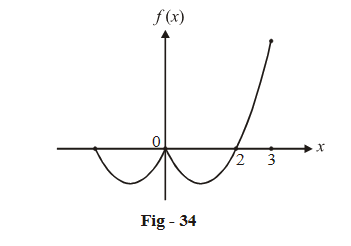Now we must understand what the definition of g(x) means.

Consider the upper definition of g(x):

$$g(x) = Min\{ f(t): - 2 \le t \le x,\,\,\,\, - 2 \le x < 0\}$$

To evaluate $$g(x)$$  at any x, we scan the entire interval from –2 to x, (this is what the variable t is for), select that value of f(t) which is minimum in this interval; this minimum value of f(t) becomes the value of the function at x.

The figure below illustrates this graphically for four different values of x (we are considering the interval $$- 2 \le x < 0,$$  the upper definition of g(x)):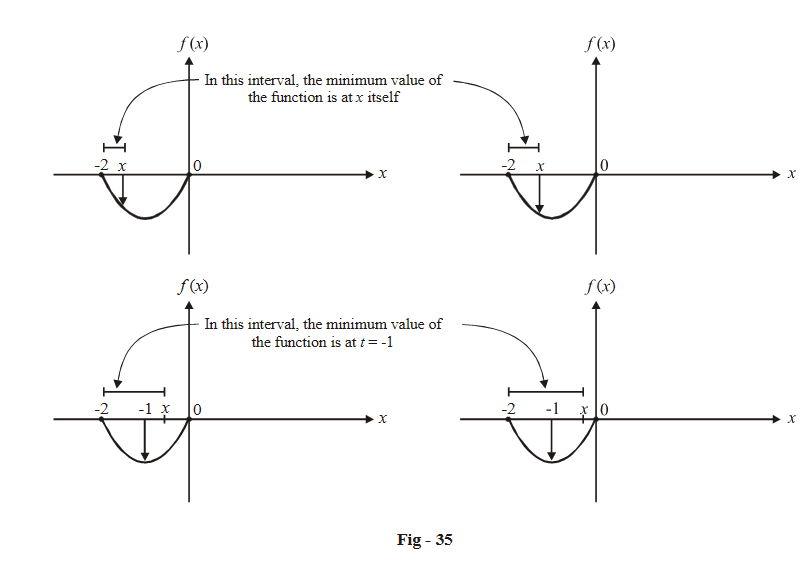Notice that where x crosses –1 (when –1 < x < 0), the minimum value of the function in the interval [–2, x] becomes fixed at t = –1. When –2 < x < –1, the minimum value of the function in the interval [–2, x] is at t = x.

So, how do we draw the graph of g (x)? In [–2, –1], the graph of g (x) will the same as f (x) (because the minimum value of f (x) is at t = x itself, as described above). In [–1, 0], the minimum value becomes fixed at t =  –1, equal to –1, so that in this interval the graph of g(x) is constant;   g (x) = 1 for $$x \in \left[ { - 1,0} \right]$$ . The graph of g (x) for $$x \in \left[ { - 2,0} \right]$$  is sketched below;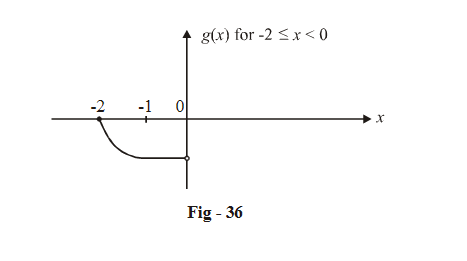For $$0 \le x \le 3,$$  the definition is $$g\left( x \right) = {\rm{Max}}\left\{ {f\left( t \right);0 \le t \le x,0 \le x \le 3} \right\}$$ . The figure below illustrates how to obtain g(x) in this case for four different values of x: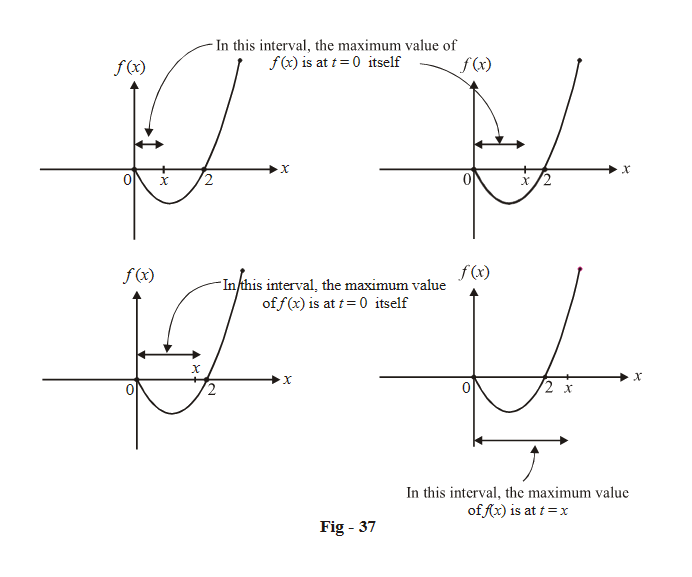Notice then untill x lies in the interval [0, 2], the maximum value of f(x) in the interval [0, x] is at   t = 0, equal to 0.

As soon as x becomes greater than 2, the maximum value of f (x) in the interval [0, x] is now at t = x.

The graph of g(x) for the interval [0, 3] is sketched below: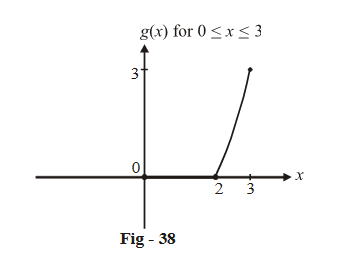The overall graph for g (x) is therefore: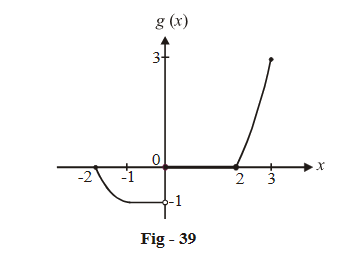We see that g (x) is discontinuous at x = 0 and non-differentiable at x =  0, 2.

Try to draw the following graphs on your own:

(i) $$f\left( x \right) = \max \left\{ {2x - {x^2};0 \le t \le x,0 \le x \le 2} \right\}$$

(ii) $$f\left( x \right) = \min \left\{ {\left| {{x^2} - 1} \right|; - 2 \le t \le x, - 2 \le x \le 2} \right\}$$

Learn from the best math teachers and top your exams

• Live one on one classroom and doubt clearing
• Practice worksheets in and after class for conceptual clarity
• Personalized curriculum to keep up with school